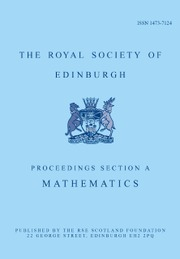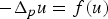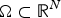Home
Hostname: page-component-65d66dc8c9-f7c9f Total loading time: 0.622 Render date: 2021-09-28T21:11:43.262Z Has data issue: true Feature Flags: { "shouldUseShareProductTool": true, "shouldUseHypothesis": true, "isUnsiloEnabled": true, "metricsAbstractViews": false, "figures": true, "newCiteModal": false, "newCitedByModal": true, "newEcommerce": true, "newUsageEvents": true }Proceedings of the Royal Society of Edinburgh Section A: Mathematics

# On a property of the nodal set of least energy sign-changing solutions for quasilinear elliptic equations

Published online by Cambridge University Press:  15 January 2019

## Abstract

In this note, we prove the Payne-type conjecture about the behaviour of the nodal set of least energy sign-changing solutions for the equation$-\Delta _p u = f(u)$ in bounded Steiner symmetric domains$\Omega \subset {{\open R}^N}$ under the zero Dirichlet boundary conditions. The nonlinearity f is assumed to be either superlinear or resonant. In the latter case, least energy sign-changing solutions are second eigenfunctions of the zero Dirichlet p-Laplacian in Ω. We show that the nodal set of any least energy sign-changing solution intersects the boundary of Ω. The proof is based on a moving polarization argument.

Type
Research Article
Information

## Access options

Get access to the full version of this content by using one of the access options below. (Log in options will check for institutional or personal access. Content may require purchase if you do not have access.)

## References

1 Aftalion, A. and Pacella, F.. Qualitative properties of nodal solutions of semilinear elliptic equations in radially symmetric domains. Comptes Rendus Mathematique 339 (2004), 339344 (doi: 10.1016/j.crma.2004.07.004).CrossRefGoogle Scholar
2Alessandrini, G.. Nodal lines of eigenfunctions of the fixed membrane problem in general convex domains. Commentarii Mathematici Helvetici 69 (1994), 142154 (doi: 10.1007/BF02564478).CrossRefGoogle Scholar
3Anoop, T. V., Drábek, P. and Sasi, S.. On the structure of the second eigenfunctions of the p-Laplacian on a ball. Proc. Am. Math. Soc. 144 (2016), 25032512 (doi: 10.1090/proc/12902).CrossRefGoogle Scholar
4Bartsch, T., Weth, T. and Willem, M.. Partial symmetry of least energy nodal solutions to some variational problems. J. d'Analyse Mathématique 96 (2005), 118 (doi: 10.1007/bf02787822).CrossRefGoogle Scholar
5Bobkov, V. E.. On existence of nodal solution to elliptic equations with convex-concave nonlinearities. Ufa Mathematical Journal 5 (2013), 1830 (doi: 10.13108/2013-5-2-18).CrossRefGoogle Scholar
6Bobkov, V. and Kolonitskii, S.. On qualitative properties of solutions for elliptic problems with the p-Laplacian through domain perturbations. (2017). arXiv preprint arXiv:1701.07408.Google Scholar
7Bonheure, D., Bouchez, V., Grumiau, C. and Van Schaftingen, J.. Asymptotics and symmetries of least energy nodal solutions of Lane-Emden problems with slow growth. Commun. Contemp. Math. 10 (2008), 609631 (doi: 10.1142/S0219199708002910).CrossRefGoogle Scholar
8Brock, F. and Solynin, A.. An approach to symmetrization via polarization. Trans. Am. Math. Soc. 352 (2000), 17591796 (doi: 10.1090/s0002-9947-99-02558-1).CrossRefGoogle Scholar
9Castro, A., Cossio, J. and Neuberger, J. M.. A sign-changing solution for a superlinear Dirichlet problem. Rocky Mountain J. Math. 27 (1997), 10411053 (doi: 10.1216/rmjm/1181071858).CrossRefGoogle Scholar
10Cuesta, M., De Figueiredo, D. and Gossez, J. P.. The beginning of the Fučik spectrum for the p-Laplacian. J. Differ. Equ. 159 (1999), 212238 (doi: 10.1006/jdeq.1999.3645).CrossRefGoogle Scholar
11Cuesta, M., De Figueiredo, D. G. and Gossez, J. P.. A nodal domain property for the p-Laplacian. Comptes Rendus de l'Académie des Sciences-Series I-Mathematics 330 (2000), 669673 (doi: 10.1016/S0764-4442(00)00245-7).CrossRefGoogle Scholar
12Damascelli, L.. On the nodal set of the second eigenfunction of the laplacian in symmetric domains in${\open {R}}^N$. Atti della Accademia Nazionale dei Lincei. Classe di Scienze Fisiche, Matematiche e Naturali. Rendiconti Lincei. Matematica e Applicazioni 11 (2000), 175181, http://eudml.org/doc/252373.Google Scholar
13Drábek, P. and Robinson, S. B.. Resonance problems for the p-Laplacian. J. Funct. Anal. 169 (1999), 189200 (doi: 10.1006/jfan.1999.3501).CrossRefGoogle Scholar
14Drábek, P., Kufner, A. and Nicolosi, F.. Quasilinear elliptic equations with degenerations and singularities, vol. 5 (Berlin: Walter de Gruyter, 1997), (doi: 10.1515/9783110804775).CrossRefGoogle Scholar
15Fournais, S.. The nodal surface of the second eigenfunction of the Laplacian in${\open {R}}^D$ can be closed. J. Differ. Equ. 173 (2001), 145159 (doi: 10.1006/jdeq.2000.3868).CrossRefGoogle Scholar
16Ghoussoub, N.. Duality and perturbation methods in critical point theory vol. 107 (Cambridge: Cambridge University Press, 1993).CrossRefGoogle Scholar
17Grossi, M., Grumiau, C. and Pacella, F.. Lane-Emden problems: Asymptotic behavior of low energy nodal solutions. Annales de l'Institut Henri Poincare (C) Non Linear Analysis 30 (2013), 121140 (doi: 10.1016/j.anihpc.2012.06.005).CrossRefGoogle Scholar
18Grumiau, C. and Parini, E.. On the asymptotics of solutions of the Lane-Emden problem for the p-Laplacian. Archiv der Mathematik 91 (2008), 354365 (doi: 10.1007/s00013-008-2854-y).CrossRefGoogle Scholar
19Grumiau, C. and Troestler, C.. Nodal line structure of least energy nodal solutions for Lane-Emden problems. Comptes Rendus Mathematique 347 (2009), 767771 (doi: 10.1016/j.crma.2009.04.023).CrossRefGoogle Scholar
20Hoffmann-Ostenhof, M., Hoffmann-Ostenhof, T. and Nadirashvili, N.. The nodal line of the second eigenfunction of the Laplacian in${\open {R}}^2$ can be closed. Duke Math. J. 90 (1997), 631640 (doi: 10.1215/S0012-7094-97-09017-7).CrossRefGoogle Scholar
21Kawohl, B.. Rearrangements and convexity of level sets in PDE. Lecture notes in mathematics, vol. 1150 (Berlin, Heidelberg: Springer, 1985 (doi: 10.1007/BFb0075060).CrossRefGoogle Scholar
22Lieberman, G. M.. Boundary regularity for solutions of degenerate elliptic equations. Nonlinear. Anal. Theory. Methods. Appl. 12 (1988), 12031219 (doi: 10.1016/0362-546x(88)90053-3).CrossRefGoogle Scholar
23Nazarov, A. I.. On Solutions to the Dirichlet problem for an equation with p-Laplacian in a spherical layer. Proc. St. Petersburg Math. Soc. 10 (2004), 3362 (doi: 10.1090/trans2/214/03).Google Scholar
24Payne, L. E.. Isoperimetric inequalities and their applications. SIAM Rev. 9 (1967), 453488 (doi: 10.1137/1009070).CrossRefGoogle Scholar
25Payne, L. E.. On two conjectures in the fixed membrane eigenvalue problem. Zeitschrift für angewandte Mathematik und Physik 24 (1973), 721729 (doi: 10.1007/BF01597076).CrossRefGoogle Scholar
26Serrin, J.. A symmetry problem in potential theory. Arch. Ration. Mech. Anal. 43 (1971), 304318 (doi: 10.1007/BF00250468).CrossRefGoogle Scholar
27Tolksdorf, P.. Regularity for a more general class of quasilinear elliptic equations. J. Differ. Equ. 51 (1984), 126150 (doi: 10.1016/0022-0396(84)90105-0).CrossRefGoogle Scholar
28Vázquez, J. L.. A strong maximum principle for some quasilinear elliptic equations. Appl. Math. Optim. 12 (1984), 191202 (doi: 10.1007/bf01449041).CrossRefGoogle Scholar

# Send article to Kindle

Note you can select to send to either the @free.kindle.com or @kindle.com variations. ‘@free.kindle.com’ emails are free but can only be sent to your device when it is connected to wi-fi. ‘@kindle.com’ emails can be delivered even when you are not connected to wi-fi, but note that service fees apply.

Find out more about the Kindle Personal Document Service.

On a property of the nodal set of least energy sign-changing solutions for quasilinear elliptic equations
Available formats
×

# Send article to Dropbox

To send this article to your Dropbox account, please select one or more formats and confirm that you agree to abide by our usage policies. If this is the first time you use this feature, you will be asked to authorise Cambridge Core to connect with your <service> account. Find out more about sending content to Dropbox.

On a property of the nodal set of least energy sign-changing solutions for quasilinear elliptic equations
Available formats
×

# Send article to Google Drive

To send this article to your Google Drive account, please select one or more formats and confirm that you agree to abide by our usage policies. If this is the first time you use this feature, you will be asked to authorise Cambridge Core to connect with your <service> account. Find out more about sending content to Google Drive.

On a property of the nodal set of least energy sign-changing solutions for quasilinear elliptic equations
Available formats
×
×